cancel
Showing results for
Did you mean:Regular Visitor

## DAX currency translation

Hi,

I would be really greatful is someone could assist.  I'm very new to power BI and after searching a lot I'm still struggling for a solution here that suits my setup.

My data is all in one currency & I want to convert it into different versions of dollar rates.  The DAX query i pulled together worked okish when I had the same rate for each period (I dont have dates in the data, just numbered financial periods 1 to 12).

However some of my currency scenarios have a different rate per period and they pull in blank, so I need help changing this query to take into account the rates at different periods.

The second issue is in my value column I'm converting there is also volume that I do no want to convert and keep at the same value, this pulls from my P&L column in the data sheet

Current DAX formula:

var value_ = CALCULATE(DATASHEETS[Sum of Value])
var ExchangeRate_ = selectedvalue('Currency'[Currency rate])
return
value_ * ExchangeRate_

Data sheet: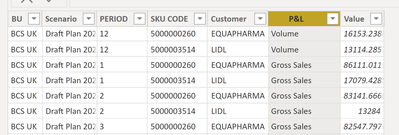Currency sheet:Also including relationships if this helps - the Period reference is what links them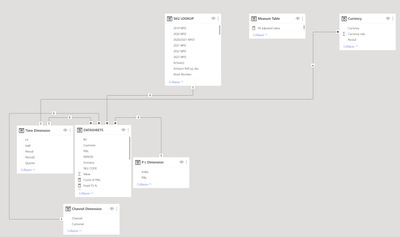Thanks for any assistance

1 ACCEPTED SOLUTIONImpactful Individual

ok i duplicated the values so i can have a similar scenario:
im still not sure if i understood correctly but here is my new table i sampled randome data numbers: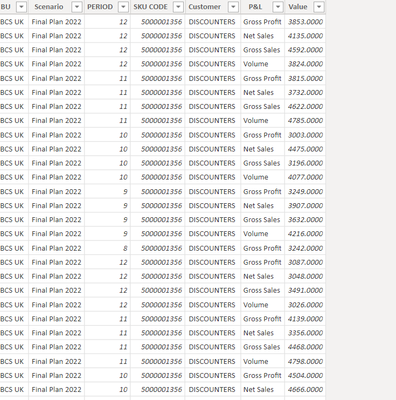as you see  i have multiple gross profit on period 9 or 12 or whatever: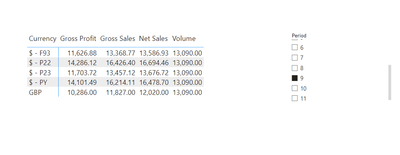``sum of values = CALCULATE(SUM(Daatasheet[Value]),ALL(Daatasheet[Value]))``

now use the same formula but use:

``Correct amount = IF(FIRSTNONBLANK(Daatasheet[P&L],1)<>"Volume",SUMX('Currency','Currency'[Currency rate] *[valueis]),SUM(Daatasheet[Value]))``
23 REPLIES 23Super User

Hi @Glen_
Kindly refer to attached file with the proposed solution. I hope this is what you're looking for.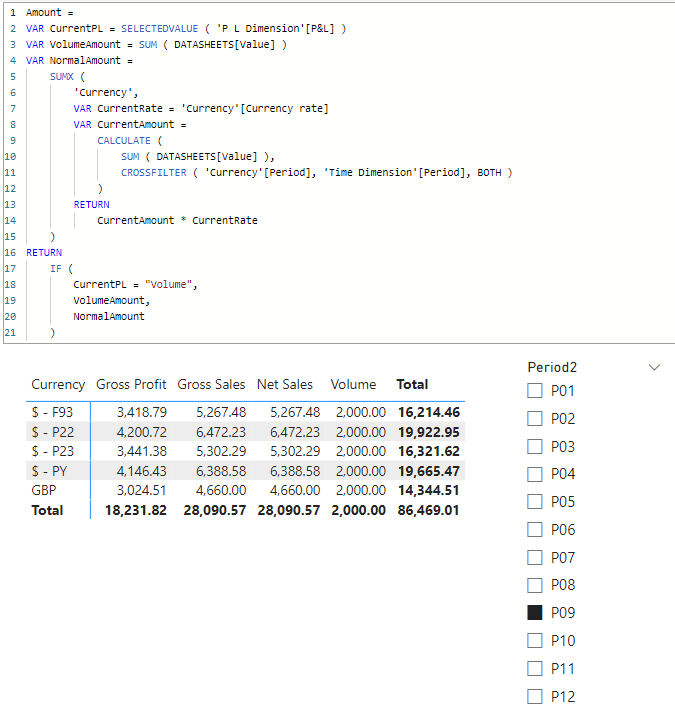``````Amount =
VAR CurrentPL = SELECTEDVALUE ( 'P L Dimension'[P&L] )
VAR VolumeAmount = SUM ( DATASHEETS[Value] )
VAR NormalAmount =
SUMX (
'Currency',
VAR CurrentRate = 'Currency'[Currency rate]
VAR CurrentAmount =
CALCULATE (
SUM ( DATASHEETS[Value] ),
CROSSFILTER ( 'Currency'[Period], 'Time Dimension'[Period], BOTH )
)
RETURN
CurrentAmount * CurrentRate
)
RETURN
IF (
CurrentPL = "Volume",
VolumeAmount,
NormalAmount
)``````Impactful Individual

Hello @Glen_

Can you please provide some data sample and an example where it went wrongRegular Visitor

No problem, I've added a sample of the data below

Also - screenshot of the results (from a more complete set):

Volume figures are having fx changes applied- need these to stay the same as they are not impacted by currency rates, GBP vols are correct as a rate of 1 is being applied.

Only 3 currencies are pulling in (the ones that have exactly the same rate each period).  "\$ - PY" & "\$ - F93" are not and they vary by periodDATASHEETS:

 BU Scenario PERIOD SKU CODE Customer P&L Value BCS UK Final Plan 2022 12 5000001356 DISCOUNTERS Gross Profit 3024.5126 BCS UK Final Plan 2022 12 5000001356 DISCOUNTERS Net Sales 4660 BCS UK Final Plan 2022 12 5000001356 DISCOUNTERS Gross Sales 4660 BCS UK Final Plan 2022 12 5000001356 DISCOUNTERS Volume 2000 BCS UK Final Plan 2022 11 5000001356 DISCOUNTERS Gross Profit 3024.5126 BCS UK Final Plan 2022 11 5000001356 DISCOUNTERS Net Sales 4660 BCS UK Final Plan 2022 11 5000001356 DISCOUNTERS Gross Sales 4660 BCS UK Final Plan 2022 11 5000001356 DISCOUNTERS Volume 2000 BCS UK Final Plan 2022 10 5000001356 DISCOUNTERS Gross Profit 3024.5126 BCS UK Final Plan 2022 10 5000001356 DISCOUNTERS Net Sales 4660 BCS UK Final Plan 2022 10 5000001356 DISCOUNTERS Gross Sales 4660 BCS UK Final Plan 2022 10 5000001356 DISCOUNTERS Volume 2000 BCS UK Final Plan 2022 9 5000001356 DISCOUNTERS Gross Profit 3024.5126 BCS UK Final Plan 2022 9 5000001356 DISCOUNTERS Net Sales 4660 BCS UK Final Plan 2022 9 5000001356 DISCOUNTERS Gross Sales 4660 BCS UK Final Plan 2022 9 5000001356 DISCOUNTERS Volume 2000 BCS UK Final Plan 2022 8 5000001356 DISCOUNTERS Gross Profit 3024.5126

Currency:

 Period Currency Currency rate 1 GBP 1 2 GBP 1 3 GBP 1 4 GBP 1 5 GBP 1 6 GBP 1 7 GBP 1 8 GBP 1 9 GBP 1 10 GBP 1 11 GBP 1 12 GBP 1 1 \$ - P22 1.38889 2 \$ - P22 1.38889 3 \$ - P22 1.38889 4 \$ - P22 1.38889 5 \$ - P22 1.38889 6 \$ - P22 1.38889 7 \$ - P22 1.38889 8 \$ - P22 1.38889 9 \$ - P22 1.38889 10 \$ - P22 1.38889 11 \$ - P22 1.38889 12 \$ - P22 1.38889 1 \$ - F93 1.35583 2 \$ - F93 1.34944 3 \$ - F93 1.3132 4 \$ - F93 1.28341 5 \$ - F93 1.24614 6 \$ - F93 1.22784 7 \$ - F93 1.20292 8 \$ - F93 1.18881 9 \$ - F93 1.13036 10 \$ - F93 1.137829991 11 \$ - F93 1.137829991 12 \$ - F93 1.137829991 1 \$ - P23 1.137829991 2 \$ - P23 1.137829991 3 \$ - P23 1.137829991 4 \$ - P23 1.137829991 5 \$ - P23 1.137829991 6 \$ - P23 1.137829991 7 \$ - P23 1.137829991 8 \$ - P23 1.137829991 9 \$ - P23 1.137829991 10 \$ - P23 1.137829991 11 \$ - P23 1.137829991 12 \$ - P23 1.137829991 1 \$ - PY 1.36521 2 \$ - PY 1.3929 3 \$ - PY 1.38469 4 \$ - PY 1.38592 5 \$ - PY 1.41457 6 \$ - PY 1.39748 7 \$ - PY 1.38329 8 \$ - PY 1.37772 9 \$ - PY 1.37094 10 \$ - PY 1.36657 11 \$ - PY 1.33914 12 \$ - PY 1.33169

Thanks againImpactful Individual

hello thank you for providing me the details
im not sure i understood  you correctly but is this the result you want?

``Correct amount = IF(SELECTEDVALUE(Daatasheet[P&L]) <>"Volume",SUMX('Currency','Currency'[Currency rate] * CALCULATE(VALUES(Daatasheet[Value]))),SUM(Daatasheet[Value]))``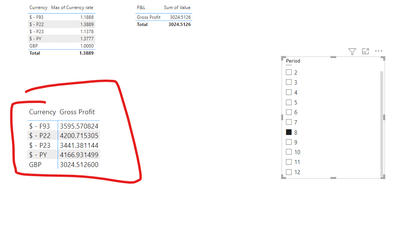Regular Visitor

I get this error when I try to recreate using that line?Looking at your screenshot, the volumes (from P&L field in datasheet) are changing - these would need to stay the same and not multiplied by fx rates?Thanks againImpactful Individual

you can also use this if you want to have multiple condition :

``Correct amount = IF(AND( SELECTEDVALUE(Daatasheet[P&L])<>"Volume",SELECTEDVALUE(Daatasheet[P&L])<>"Gross Profit"),SUMX('Currency','Currency'[Currency rate] * CALCULATE(SELECTEDVALUE(Daatasheet[Value]))),SUM(Daatasheet[Value]))``Impactful Individual

can you show me what you have in the table? and i reread the issue and edited my post please see if this is the result you wantRegular Visitor

Hi

I've updated to match yours, but still get this message. No error message when saving the formula - but no luck calculating the table

Could this be because Period number is used multiple times in the datasheet, along with the currency sheet?

fxv2 = if(SELECTEDVALUE(DATASHEETS[P&L]) <>"Volume",SUMX('Currency','Currency'[Currency rate] * CALCULATE(VALUES(DATASHEETS[Value]))),SUM(DATASHEETS[Value]))@eliasayyImpactful Individual

if this still doesnt work replace it with firstnonblank(datasheet[value],0)

so uou get something like

``Correct amount = IF(FIRSTNONBLANK(Daatasheet[P&L],0)<>"Volume",SUMX('Currency','Currency'[Currency rate] * CALCULATE(FIRSTNONBLANK(Daatasheet[Value],0))),SUM(Daatasheet[Value]))``Regular Visitor

with selected value - volumes now pull in and are all the same which is good, but none of the other P&L lines are coming in:

fxv2 = if(SELECTEDVALUE(DATASHEETS[P&L]) <>"Volume",SUMX('Currency','Currency'[Currency rate] * CALCULATE(SELECTEDVALUE(DATASHEETS[Value]))),SUM(DATASHEETS[Value]))Thanks againImpactful Individual

if this still doesnt work do you think you can share your powerbi file?
if no provide a full page of all filter and slicers you might be usingImpactful Individual

ok try

``Correct amount = IF(FIRSTNONBLANK(Daatasheet[P&L],0)<>"Volume",SUMX('Currency','Currency'[Currency rate] * CALCULATE(FIRSTNONBLANK(Daatasheet[Value],0))),SUM(Daatasheet[Value]))``Regular Visitor

I get numbers now in for the other P&L lines - but much lower than they should be:

This was before (where i could only pull in where the rate was the same for every period.  You can see positive figures & much higher values: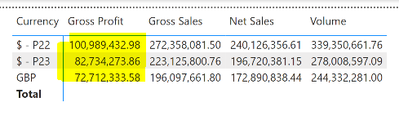with this solution, the numbers are negative and very low.  The volume is correct thoughRevisiting the model - its linked to the time dimension, as I think DATASHEETS have multiple different results for each period unlike Time Dimension:  Sample below, could this help?Time Dimension

 Period Period2 Quarter FY Half 1 P01 Q1 FY H1 2 P02 Q1 FY H1 3 P03 Q1 FY H1 4 P04 Q2 FY H1 5 P05 Q2 FY H1 6 P06 Q2 FY H1 7 P07 Q3 FY H2 8 P08 Q3 FY H2 9 P09 Q3 FY H2 10 P10 Q4 FY H2 11 P11 Q4 FY H2 12 P12 Q4 FY H2

ThanksImpactful Individual

does your values for example gross profit has multiple values in the same period?
say

 Period P&L Value 1 gross profit -10,000 1 gross profit -4,000 1 gross profit 20,000Regular Visitor

Thats correct it does, the data is for multiple customers & they sometimes take the same products so there will be many thousand rows with say period 1 and gross profit and a different value figureImpactful Individual

ok this is why its giving you the numebrs the formula adds all period 1 gross profit so can you please tell me exactly how you want to filter it out?Regular Visitor

Sure, so I'm looking to be able to slice between the different currencies.

If no other slices / filters are on I would be looking for everything to total Period 1 to 12 applying the individual rate for each period to every line in the datasheet (except volume), and pull the results depending on which currency rate is selected in the filters.

Thanks againImpactful Individual

ok i duplicated the values so i can have a similar scenario:
im still not sure if i understood correctly but here is my new table i sampled randome data numbers:as you see  i have multiple gross profit on period 9 or 12 or whatever:``sum of values = CALCULATE(SUM(Daatasheet[Value]),ALL(Daatasheet[Value]))``

now use the same formula but use:

``Correct amount = IF(FIRSTNONBLANK(Daatasheet[P&L],1)<>"Volume",SUMX('Currency','Currency'[Currency rate] *[valueis]),SUM(Daatasheet[Value]))``Regular Visitor

Thats done it, thank you so much for taking all this time to help me & sorry if I didn't explain it the best to start with, again I'm very new to it all.

Have a great dayImpactful Individual

im glad i could help my friend its okay we all were once newbies